# Long Division Method to find Square root with Examples

• Last Updated : 26 Nov, 2021

Given an integer X which is a perfect square, the task is to find the square root of it by using the long division method.

Examples:

Input: N = 484
Output: 22
222 = 484

Input: N = 144
Output: 12
122 = 144

Approach:
Long division is a very common method to find the square root of a number. The following is the stepwise solution for this method:

1. Divide the digits of the number into pairs of segments starting with the digit in the units place. Let’s identify each pair and the remaining final digit(in case there is an odd count of digits in the number) as a segment.
For example:

`1225 is divided as (12 25)`2. After dividing the digits into segments, start from the leftmost segment. The largest number whose square is equal to or just less than the first segment is taken as the divisor and also as the quotient (so that the product is the square).
For example:

`9 is the closest perfect square to 12, the first segment 12`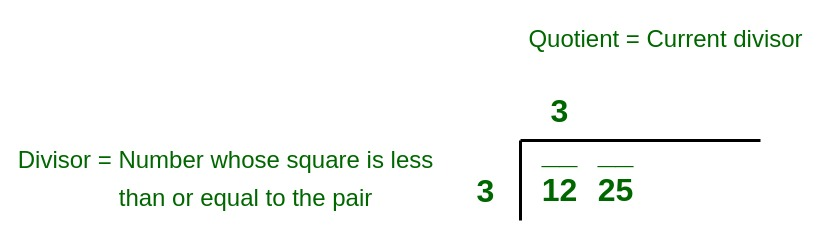3. Subtract the square of the divisor from the first segment and bring down the next segment to the right of the remainder to get the new dividend.
For example:

```12 - 9 = 3 is concatenated with next segment 25.
New dividend = 325 ```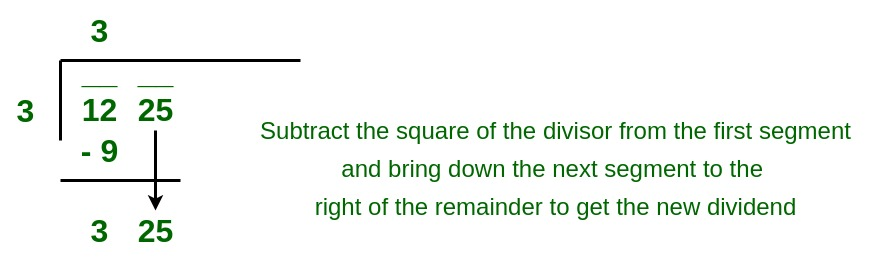4. Now, the new divisor is obtained by taking two times the previous quotient(which was 3 in the above example as 32 = 9) and concatenating it with a suitable digit which is also taken as the next digit of the quotient, chosen in such a way that the product of the new divisor and this digit is equal to, or just less than the new dividend.
For example:

`Two times quotient 3 is 6.`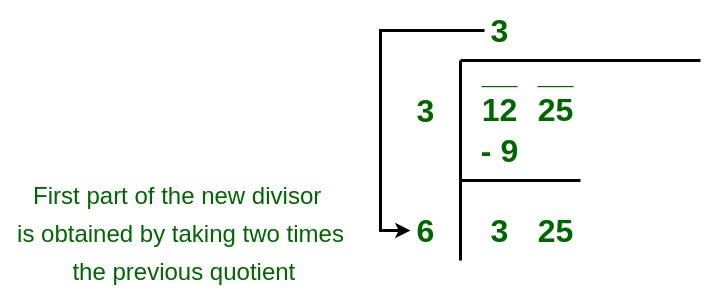`65 times 5 is 325 which is closest to the new dividend. `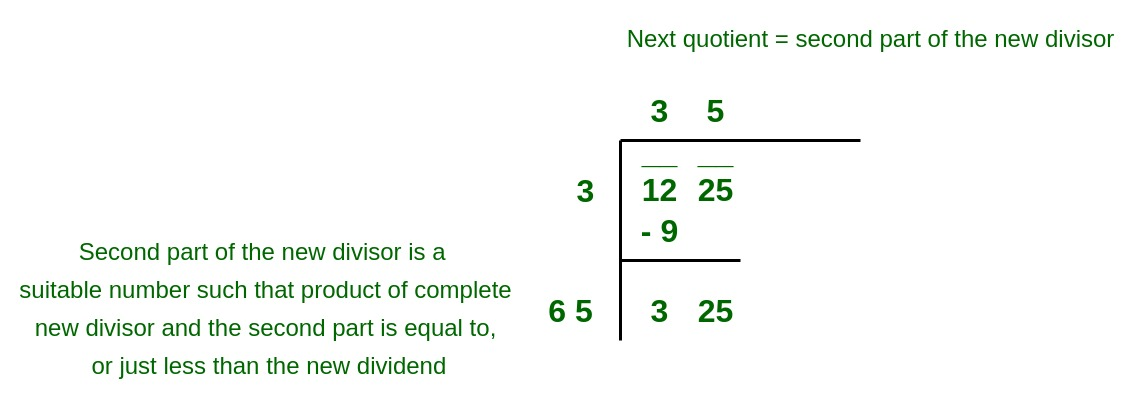5. Repeat steps (2), (3) and (4) till all the segments have been taken up. Now, the quotient so obtained is the required square root of the given number.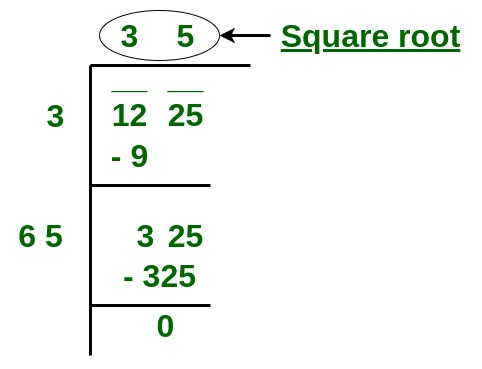Below is the implementation of the above approach:

## CPP

 `// C++ program to find the square root of a``// number by using long division method` `#include ``using` `namespace` `std;``#define INFINITY_ 9999999` `// Function to find the square root of``// a number by using long division method``int` `sqrtByLongDivision(``int` `n)``{``    ``int` `i = 0, udigit, j; ``// Loop counters``    ``int` `cur_divisor = 0;``    ``int` `quotient_units_digit = 0;``    ``int` `cur_quotient = 0;``    ``int` `cur_dividend = 0;``    ``int` `cur_remainder = 0;``    ``int` `a = { 0 };` `    ``// Dividing the number into segments``    ``while` `(n > 0) {``        ``a[i] = n % 100;``        ``n = n / 100;``        ``i++;``    ``}` `    ``// Last index of the array of segments``    ``i--;` `    ``// Start long division from the last segment(j=i)``    ``for` `(j = i; j >= 0; j--) {` `        ``// Initialising the remainder to the maximum value``        ``cur_remainder = INFINITY_;``        ``// Including the next segment in new dividend``        ``cur_dividend = cur_dividend * 100 + a[j];` `        ``// Loop to check for the perfect square``        ``// closest to each segment``        ``for` `(udigit = 0; udigit <= 9; udigit++) {` `            ``// This condition is to find the``            ``// divisor after adding a digit``            ``// in the range 0 to 9``            ``if` `(cur_remainder >= cur_dividend``                                     ``- ((cur_divisor * 10 + udigit)``                                        ``* udigit)``                ``&& cur_dividend``                           ``- ((cur_divisor * 10 + udigit) * udigit)``                       ``>= 0) {` `                ``// Calculating the remainder``                ``cur_remainder = cur_dividend - ((cur_divisor * 10``                                                 ``+ udigit)``                                                ``* udigit);` `                ``// Updating the units digit of the quotient``                ``quotient_units_digit = udigit;``            ``}``        ``}` `        ``// Adding units digit to the quotient``        ``cur_quotient = cur_quotient * 10``                       ``+ quotient_units_digit;` `        ``// New divisor is two times quotient``        ``cur_divisor = cur_quotient * 2;` `        ``// Including the remainder in new dividend``        ``cur_dividend = cur_remainder;``    ``}` `    ``return` `cur_quotient;``}` `// Driver code``int` `main()``{``    ``int` `x = 1225;``    ``cout << sqrtByLongDivision(x) << endl;``    ``return` `0;``}`

## Java

 `// Java program to find the square root of a``// number by using long division method``import` `java.util.*;` `class` `GFG{``static` `final` `int` `INFINITY_ =``9999999``;`` ` `// Function to find the square root of``// a number by using long division method``static` `int` `sqrtByLongDivision(``int` `n)``{``    ``int` `i = ``0``, udigit, j; ``// Loop counters``    ``int` `cur_divisor = ``0``;``    ``int` `quotient_units_digit = ``0``;``    ``int` `cur_quotient = ``0``;``    ``int` `cur_dividend = ``0``;``    ``int` `cur_remainder = ``0``;``    ``int` `a[] = ``new` `int``[``10``];`` ` `    ``// Dividing the number into segments``    ``while` `(n > ``0``) {``        ``a[i] = n % ``100``;``        ``n = n / ``100``;``        ``i++;``    ``}`` ` `    ``// Last index of the array of segments``    ``i--;`` ` `    ``// Start long division from the last segment(j=i)``    ``for` `(j = i; j >= ``0``; j--) {`` ` `        ``// Initialising the remainder to the maximum value``        ``cur_remainder = INFINITY_;``        ``// Including the next segment in new dividend``        ``cur_dividend = cur_dividend * ``100` `+ a[j];`` ` `        ``// Loop to check for the perfect square``        ``// closest to each segment``        ``for` `(udigit = ``0``; udigit <= ``9``; udigit++) {`` ` `            ``// This condition is to find the``            ``// divisor after adding a digit``            ``// in the range 0 to 9``            ``if` `(cur_remainder >= cur_dividend``                                     ``- ((cur_divisor * ``10` `+ udigit)``                                        ``* udigit)``                ``&& cur_dividend``                           ``- ((cur_divisor * ``10` `+ udigit) * udigit)``                       ``>= ``0``) {`` ` `                ``// Calculating the remainder``                ``cur_remainder = cur_dividend - ((cur_divisor * ``10``                                                 ``+ udigit)``                                                ``* udigit);`` ` `                ``// Updating the units digit of the quotient``                ``quotient_units_digit = udigit;``            ``}``        ``}`` ` `        ``// Adding units digit to the quotient``        ``cur_quotient = cur_quotient * ``10``                       ``+ quotient_units_digit;`` ` `        ``// New divisor is two times quotient``        ``cur_divisor = cur_quotient * ``2``;`` ` `        ``// Including the remainder in new dividend``        ``cur_dividend = cur_remainder;``    ``}`` ` `    ``return` `cur_quotient;``}`` ` `// Driver code``public` `static` `void` `main(String[] args)``{``    ``int` `x = ``1225``;``    ``System.out.print(sqrtByLongDivision(x) +``"\n"``);``}``}` `// This code is contributed by Rajput-Ji`

## Python3

 `# Python3 program to find the square root of a``# number by using long division method``INFINITY_ ``=` `9999999` `# Function to find the square root of``# a number by using long division method``def` `sqrtByLongDivision(n):``    ``i ``=` `0``    ``udigit, j ``=` `0``, ``0` `# Loop counters``    ``cur_divisor ``=` `0``    ``quotient_units_digit ``=` `0``    ``cur_quotient ``=` `0``    ``cur_dividend ``=` `0``    ``cur_remainder ``=` `0``    ``a ``=` `[``0``]``*``10` `    ``# Dividing the number into segments``    ``while` `(n > ``0``):``        ``a[i] ``=` `n ``%` `100``        ``n ``=` `n ``/``/` `100``        ``i ``+``=` `1` `    ``# Last index of the array of segments``    ``i ``-``=` `1` `    ``# Start long division from the last segment(j=i)``    ``for` `j ``in` `range``(i, ``-``1``, ``-``1``):` `        ``# Initialising the remainder to the maximum value``        ``cur_remainder ``=` `INFINITY_``        ` `        ``# Including the next segment in new dividend``        ``cur_dividend ``=` `cur_dividend ``*` `100` `+` `a[j]` `        ``# Loop to check for the perfect square``        ``# closest to each segment``        ``for` `udigit ``in` `range``(``10``):` `            ``# This condition is to find the``            ``# divisor after adding a digit``            ``# in the range 0 to 9``            ``if` `(cur_remainder >``=` `cur_dividend``                                ``-` `((cur_divisor ``*` `10` `+` `udigit)``                                ``*` `udigit)``                                ``and` `cur_dividend``                                ``-` `((cur_divisor ``*` `10` `+` `udigit) ``*` `udigit)``                                ``>``=` `0``):` `                ``# Calculating the remainder``                ``cur_remainder ``=` `cur_dividend ``-` `((cur_divisor ``*` `10``                                                ``+` `udigit)``                                                ``*` `udigit)` `                ``# Updating the units digit of the quotient``                ``quotient_units_digit ``=` `udigit`  `        ``# Adding units digit to the quotient``        ``cur_quotient ``=` `cur_quotient ``*` `10` `+` `quotient_units_digit` `        ``# New divisor is two times quotient``        ``cur_divisor ``=` `cur_quotient ``*` `2` `        ``# Including the remainder in new dividend``        ``cur_dividend ``=` `cur_remainder` `    ``return` `cur_quotient` `# Driver code` `x ``=` `1225``print``(sqrtByLongDivision(x))` `# This code is contributed by mohit kumar 29   `

## C#

 `// C# program to find the square root of a``// number by using long division method``using` `System;` `class` `GFG``{``static` `readonly` `int` `INFINITY_ =9999999;` `// Function to find the square root of``// a number by using long division method``static` `int` `sqrtBylongDivision(``int` `n)``{``    ``int` `i = 0, udigit, j; ``// Loop counters``    ``int` `cur_divisor = 0;``    ``int` `quotient_units_digit = 0;``    ``int` `cur_quotient = 0;``    ``int` `cur_dividend = 0;``    ``int` `cur_remainder = 0;``    ``int` `[]a = ``new` `int``;` `    ``// Dividing the number into segments``    ``while` `(n > 0) {``        ``a[i] = n % 100;``        ``n = n / 100;``        ``i++;``    ``}` `    ``// Last index of the array of segments``    ``i--;` `    ``// Start long division from the last segment(j=i)``    ``for` `(j = i; j >= 0; j--) {` `        ``// Initialising the remainder to the maximum value``        ``cur_remainder = INFINITY_;``        ` `        ``// Including the next segment in new dividend``        ``cur_dividend = cur_dividend * 100 + a[j];` `        ``// Loop to check for the perfect square``        ``// closest to each segment``        ``for` `(udigit = 0; udigit <= 9; udigit++) {` `            ``// This condition is to find the``            ``// divisor after adding a digit``            ``// in the range 0 to 9``            ``if` `(cur_remainder >= cur_dividend``                                    ``- ((cur_divisor * 10 + udigit)``                                        ``* udigit)``                ``&& cur_dividend``                        ``- ((cur_divisor * 10 + udigit) * udigit)``                    ``>= 0) {` `                ``// Calculating the remainder``                ``cur_remainder = cur_dividend - ((cur_divisor * 10``                                                ``+ udigit)``                                                ``* udigit);` `                ``// Updating the units digit of the quotient``                ``quotient_units_digit = udigit;``            ``}``        ``}` `        ``// Adding units digit to the quotient``        ``cur_quotient = cur_quotient * 10``                    ``+ quotient_units_digit;` `        ``// New divisor is two times quotient``        ``cur_divisor = cur_quotient * 2;` `        ``// Including the remainder in new dividend``        ``cur_dividend = cur_remainder;``    ``}` `    ``return` `cur_quotient;``}` `// Driver code``public` `static` `void` `Main(String[] args)``{``    ``int` `x = 1225;``    ``Console.Write(sqrtBylongDivision(x) +``"\n"``);``}``}`   `// This code is contributed by Rajput-Ji`

## Javascript

 ``

Output:

`35`

Time Complexity: O((log100n)2 * 10)

Auxiliary Space: O(1)

My Personal Notes arrow_drop_up